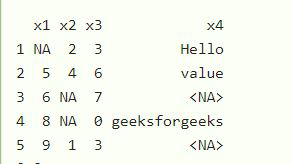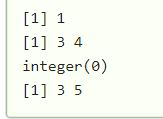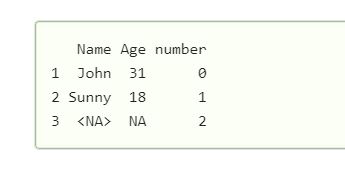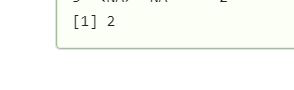Open in App
Not now

# How to check missing values in R dataframe ?

• Last Updated : 21 Apr, 2021

In this article, we are going to see how to find out the missing values in the data frame in R Programming Language.

### Approach:

Step 1: Create DataFrame.

Let us first create a data frame with some missing values and then demonstrate with an example how to find the missing values.

## R

 `data <- ``data.frame``(x1 = ``c``(``NA``, 5, 6, 8, 9),   ``                        ``x2 = ``c``(2, 4, ``NA``, ``NA``, 1), ``                        ``x3 = ``c``(3,6,7,0,3), ``                        ``x4 = ``c``(``"Hello"``, ``"value"``,``                               ``NA``, ``"geeksforgeeks"``, ``NA``)) ``                                                                            ` `display``(data)`

Output:We have created a data frame with some missing values (NA).

Step 2: Now to check the missing values we are using is.na() function in R and print out the number of missing items in the data frame as shown below.

Syntax: is.na()

Parameter: x: data frame

Example 1:

In this example, we have first created data with some missing values and then found the missing value in particular columns x1,×2, x3, and x4 respectively using the above function.

## R

 `data <- ``data.frame``(x1 = ``c``(``NA``, 5, 6, 8, 9),       ``                        ``x2 = ``c``(2, 4, ``NA``, ``NA``, 1), ``                        ``x3 = ``c``(3,6,7,0,3), ``                        ``x4 = ``c``(``"Hello"``, ``"value"``,``                               ``NA``, ``"geeksforgeeks"``, ``NA``)) ``                                                                            ` `data                    `` ` `# to find out the missing value``which``(``is.na``(data\$x1))``which``(``is.na``(data\$x2)) ``which``(``is.na``(data\$x3)) ``which``(``is.na``(data\$x4))`

Output:Print the variables which have missing values and the number of missing values.

Example 2:

Let’s find the number of missing values using a different approach, here in this example, we have created data with missing values and then find the number of missing values in the data.

## R

 `Name <- ``c``(``"John"``, ``"Sunny"``, ``NA``)``Age <- ``c``(31, 18, ``NA``)``number<- ``c``(``"0"``, ``"1"``, ``"2"``)`` ` `data <- ``data.frame``(Name, Sex, number)`` ` `display``(data)`

Output:We have a data frame, and we have to find the number of missing values in this data frame.

## R

 `Name <- ``c``(``"John"``, ``"Sunny"``, ``NA``)``Age <- ``c``(31, 18, ``NA``)``number<- ``c``(``"0"``, ``"1"``, ``"2"``)`` ` `data <- ``data.frame``(Name, Sex, number)``sum``(``is.na``(data))`

Output:My Personal Notes arrow_drop_up